# Disclaimer

All materials and contents are provided for information purposes only. Moscow Exchange assumes no obligations, and makes no representations or warranties, whether express or implied, with regard to the accuracy, completeness, quality, merchantability, correctness, compliance with any specific methodologies and descriptions or the fitness for any particular purpose as well as volume, structure, format, submission dates and timeliness, of such materials and contents. Any such materials and contents (or any portion thereof) cannot be used for any investment or commercial purposes including the creation of financial instruments, products or indices.

# Aggregated Netflow Analytics 1¶

### Description¶

The product contains netflow(difference between buy and sell volume) for each of the following groups:

• BASE_H - clients with a high number of orders
• BASE_I - clients with a high trading volume and a low number of orders
• BASE_R - the others

Netflow is calculated for the following instruments:

• SBER
• GAZP
• USDRUB
• Si
• RI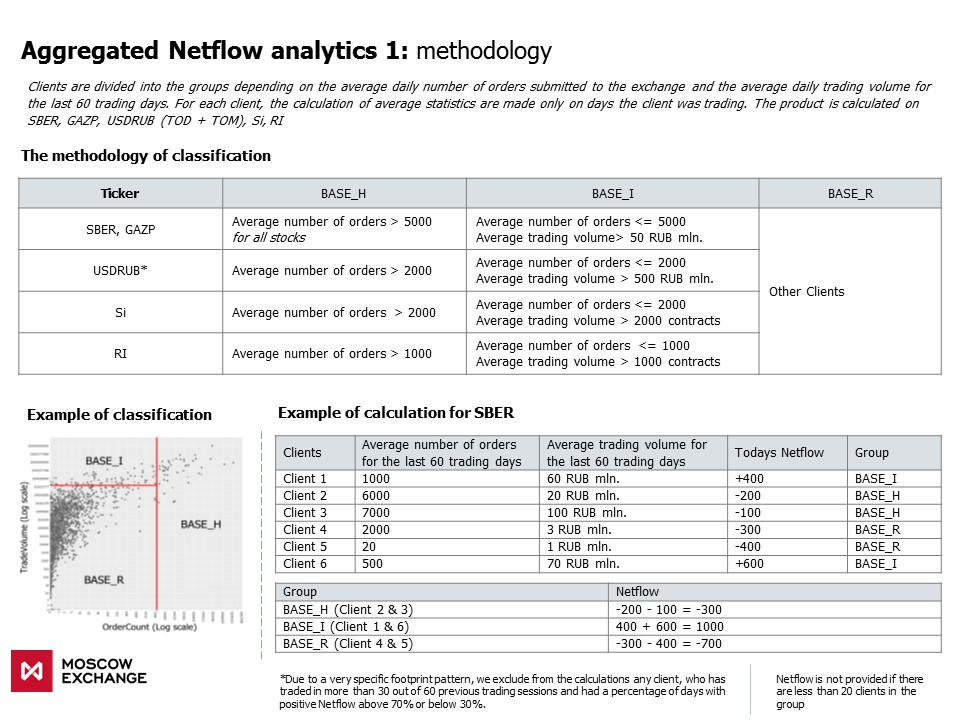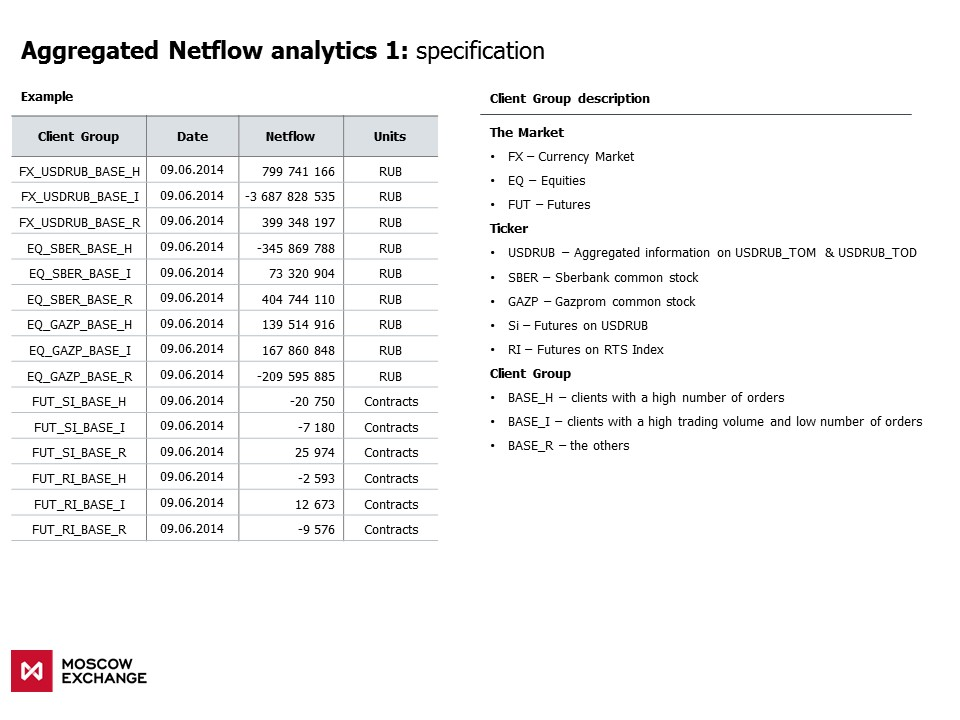In :
#libs import
import pandas as pd
import matplotlib.pyplot as plt
import seaborn as sns
import numpy as np
from scipy.stats import spearmanr

%matplotlib inline

In :
sns.set_style("dark")
plt.rcParams['figure.figsize'] = (15, 7)

# colors
graphcolors = {'red'    :'#c8102e',
'grey'   :'#51626f',
'blue'   :'#0070c0',
'orange' :'#ffa100',
'green'  :'#00b050',
'black'  :'#000000',
'white'  :'#ffffff'}


# Data Review¶

In :
df = pd.read_csv('all_instruments.csv', parse_dates = ['SESSIONID'], index_col = 'SESSIONID')

Out:
FUT_RI_BASE_H FUT_RI_BASE_I FUT_RI_BASE_R EQ_SBER_BASE_H EQ_SBER_BASE_I EQ_SBER_BASE_R FX_USDRUB_BASE_H FX_USDRUB_BASE_I FX_USDRUB_BASE_R EQ_GAZP_BASE_H EQ_GAZP_BASE_I EQ_GAZP_BASE_R FUT_SI_BASE_H FUT_SI_BASE_I FUT_SI_BASE_R
SESSIONID
2014-04-03 5347.0 -3230.0 -1919.0 -1.591269e+08 -226091118.1 3.701399e+08 NaN NaN NaN NaN NaN NaN -263.0 -26268.0 25728.0
2014-04-04 -15803.0 3885.0 12667.0 1.930270e+08 279655427.4 -5.005727e+08 -4.590551e+08 -6.625603e+09 7.846035e+08 NaN NaN NaN 71759.0 -20848.0 -50967.0
2014-04-07 30575.0 10064.0 -40456.0 -1.765621e+09 948203869.8 8.086838e+08 3.975500e+09 1.599001e+10 -2.031079e+09 NaN NaN NaN -102225.0 4135.0 94120.0
2014-04-08 196.0 -9275.0 8930.0 -6.775359e+08 -82795538.9 7.370937e+08 -1.033231e+08 1.268723e+10 5.986203e+08 NaN NaN NaN -50784.0 71946.0 -21672.0
2014-04-09 5880.0 -8116.0 2245.0 -1.041608e+09 -425282705.2 1.456435e+09 -2.093416e+09 7.414839e+09 -1.661588e+09 NaN NaN NaN 161887.0 -139912.0 -21808.0
In :
df.tail()

Out:
FUT_RI_BASE_H FUT_RI_BASE_I FUT_RI_BASE_R EQ_SBER_BASE_H EQ_SBER_BASE_I EQ_SBER_BASE_R FX_USDRUB_BASE_H FX_USDRUB_BASE_I FX_USDRUB_BASE_R EQ_GAZP_BASE_H EQ_GAZP_BASE_I EQ_GAZP_BASE_R FUT_SI_BASE_H FUT_SI_BASE_I FUT_SI_BASE_R
SESSIONID
2017-12-25 2420.0 150.0 -2436.0 6.015159e+07 248138581.2 -399688389.8 7.237792e+09 -9.401506e+09 2.969537e+09 49415915.1 -56399743.0 22589979.4 -50438.0 20343.0 26707.0
2017-12-26 797.0 1489.0 -2253.0 -9.345373e+07 155014830.0 -50272832.1 1.248377e+10 -9.966659e+09 8.445983e+09 185435087.0 -97760469.9 -51489974.6 -27318.0 -5022.0 30755.0
2017-12-27 -1370.0 6335.0 -4528.0 1.622703e+09 -826386398.8 -823850816.8 -7.142288e+08 1.135256e+10 6.675267e+08 88595922.9 -25298062.3 -60114251.0 -87277.0 -15368.0 100262.0
2017-12-28 2554.0 -2343.0 -148.0 3.369231e+08 -681576192.3 338019690.9 7.676596e+09 -8.835617e+08 7.273123e+09 -115055952.5 18421067.9 88357659.5 25801.0 -15566.0 -11545.0
2017-12-29 3364.0 -5485.0 2162.0 1.059181e+09 -547221050.3 -517989420.6 6.464532e+09 -2.034585e+10 8.562582e+08 -260019028.9 110176511.5 145941782.6 -31202.0 1360.0 28577.0

The columns names include:

1. FUT, EQ, FX - market
2. RI, SBER, USDRUB, GAZP, Si - instrument
3. BASE_H, BASE_I, BASE_R - client group

### Hypothesis testing. Is netflow correlated with price?¶

#### SBER¶

In :
cl_groups = ['BASE_H', 'BASE_I', 'BASE_R']
sber_df = pd.DataFrame(index = df.index)
for gr in cl_groups:
sber_df[gr] = df['EQ_SBER_' + gr]

Out:
BASE_H BASE_I BASE_R
SESSIONID
2014-04-03 -1.591269e+08 -226091118.1 3.701399e+08
2014-04-04 1.930270e+08 279655427.4 -5.005727e+08
2014-04-07 -1.765621e+09 948203869.8 8.086838e+08
2014-04-08 -6.775359e+08 -82795538.9 7.370937e+08
2014-04-09 -1.041608e+09 -425282705.2 1.456435e+09
In :
# reading the prices
sber_ohlc = pd.read_csv('Price_SBER.csv', index_col= 0, parse_dates = ['begin', 'end'])

Out:
open close high low value volume begin end
0 100.20 99.96 100.31 99.7 119364942.2 1194750 2014-01-06 10:00:00 2014-01-06 10:09:59
1 99.96 100.06 100.06 99.9 61075442.1 611170 2014-01-06 10:10:00 2014-01-06 10:19:59
In :
#close auction prices
close_auction = sber_ohlc[(sber_ohlc['begin'].dt.hour == 18) & (sber_ohlc['begin'].dt.minute == 30)].copy()
close_auction.begin = close_auction.begin.map(lambda x: x + pd.Timedelta(hours = -x.hour, minutes = -x.minute, seconds = -x.second))
close_auction = close_auction.set_index('begin')[['close']]
close_auction.index.name = 'SESSIONID'

Out:
close
SESSIONID
2014-01-06 98.91
2014-01-08 98.19
2014-01-09 98.00
2014-01-10 99.20
2014-01-13 100.25
In :
sber_df['close_auction_price'] = close_auction.close

Out:
BASE_H BASE_I BASE_R close_auction_price
SESSIONID
2014-04-03 -1.591269e+08 -226091118.1 3.701399e+08 81.44
2014-04-04 1.930270e+08 279655427.4 -5.005727e+08 83.03
2014-04-07 -1.765621e+09 948203869.8 8.086838e+08 79.52
2014-04-08 -6.775359e+08 -82795538.9 7.370937e+08 79.60
2014-04-09 -1.041608e+09 -425282705.2 1.456435e+09 78.90
In :
sber_df[['BASE_H', 'BASE_I', 'BASE_R']].cumsum().plot()
sber_df.close_auction_price.plot(secondary_y= True, label = 'price', color = 'orange')
plt.legend(loc = (0.005, 0.81))
plt.show()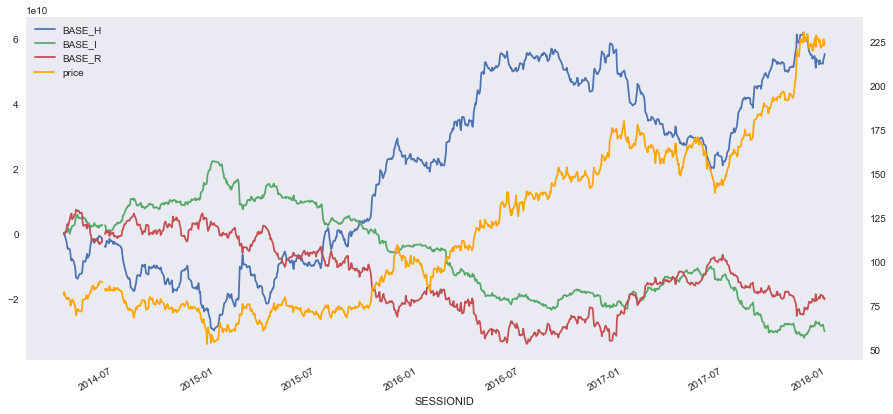We can see that there is correlation between SBER price and BASE_H

In :
sber_df['price_chng'] = sber_df.close_auction_price.pct_change()
sber_df.corr()

Out:
BASE_H BASE_I BASE_R close_auction_price price_chng
BASE_H 1.000000 -0.592876 -0.823198 -0.003475 0.602689
BASE_I -0.592876 1.000000 0.039609 -0.015994 -0.025792
BASE_R -0.823198 0.039609 1.000000 0.026627 -0.727230
close_auction_price -0.003475 -0.015994 0.026627 1.000000 0.043519
price_chng 0.602689 -0.025792 -0.727230 0.043519 1.000000

Let's examine correlation between Netflows and close-to-close next day return. For each of the BASE_H, BASE_I, BASE_R we will set up a hypothesis H0, stating that there is no correlation between next day return and Netflow. The alternative hypothesis H1 states that the correlation is not zero. We will test this hypothesis using 5% significance level.

In :
sber_df['next_price_chng'] = sber_df.price_chng.shift(-1)
sber_df = sber_df.dropna()

In :
for g in ['BASE_H', 'BASE_I', 'BASE_R']:
scor = spearmanr(sber_df[g], sber_df['next_price_chng'])
if np.abs(scor) < 0.05:
print('H0 is rejected, correlation between {0} and next day return is'.format(g),
round(scor, 3),'; p-value:', round(scor, 3))
else:
print('H0 is not rejected, correlation between {0} and next day return is'.format(g),
round(scor, 3),'; p-value:', round(scor, 3))

H0 is rejected, correlation between BASE_H and next day return is 0.091 ; p-value: 0.005
H0 is not rejected, correlation between BASE_I and next day return is -0.035 ; p-value: 0.279
H0 is rejected, correlation between BASE_R and next day return is -0.068 ; p-value: 0.037


We can reject the hypothesis H0 about zero correlation between BASE_H and SBER next day return as well as BASE_R and SBER next day return at the 5% significance level.

#### USDRUB¶

In :
cols = [x for x in df.columns if x[:6] == 'FUT_SI' or x[:2] == 'FX']
usdrub_df = df[cols].copy()

usdrub_ohlc = pd.read_csv('Price_USDRUB.csv', index_col= 0, parse_dates = ['begin', 'end'])
close_auction = usdrub_ohlc[(usdrub_ohlc['begin'].dt.hour == 18) & (usdrub_ohlc['begin'].dt.minute == 30)].copy()
close_auction.begin = close_auction.begin.map(lambda x: x + pd.Timedelta(hours = -x.hour, minutes = -x.minute, seconds = -x.second))
close_auction = close_auction.set_index('begin')[['close']]
close_auction.index.name = 'SESSIONID'
usdrub_df['close_auction_price'] = close_auction.close

In :
fx_cols = [x for x in df.columns if x[:2] == 'FX']
usdrub_df[fx_cols].cumsum().plot()
usdrub_df.close_auction_price.plot(secondary_y= True, label = 'price', color = 'orange')
plt.legend(loc = (0.005, 0.81))
plt.show()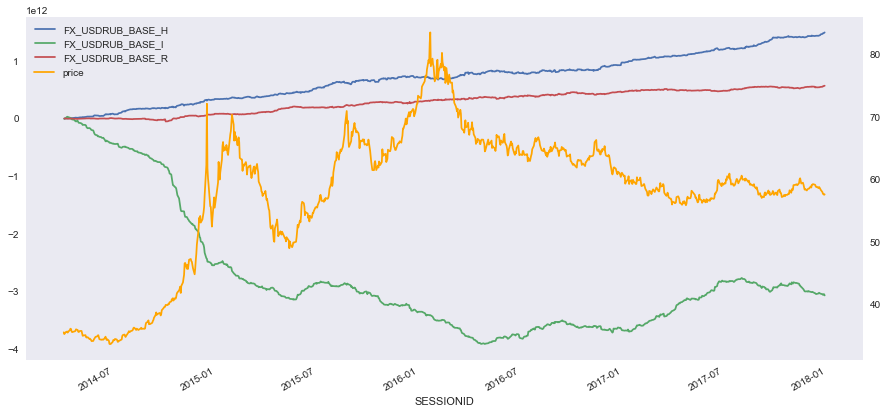In :
fut_cols = [x for x in df.columns if x[:6] == 'FUT_SI']
usdrub_df[fut_cols].cumsum().plot()
usdrub_df.close_auction_price.plot(secondary_y= True, label = 'price', color = 'orange')
plt.legend(loc = (0.005, 0.81))
plt.show()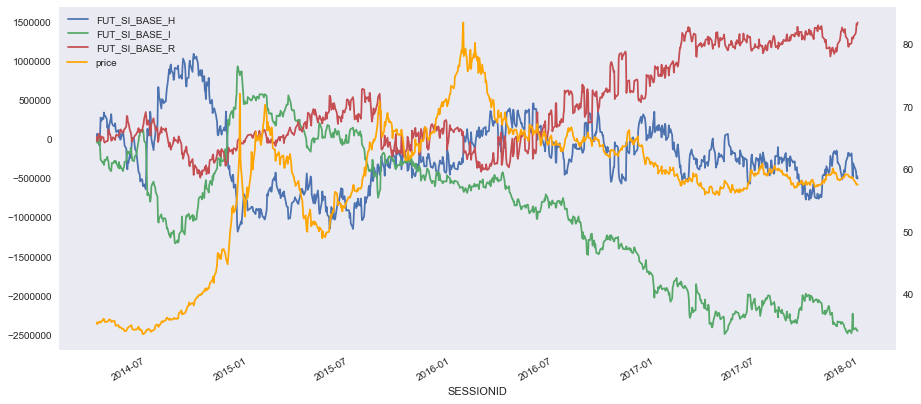In :
usdrub_df['price_chng'] = usdrub_df.close_auction_price.pct_change()
usdrub_df.corr()

Out:
FX_USDRUB_BASE_H FX_USDRUB_BASE_I FX_USDRUB_BASE_R FUT_SI_BASE_H FUT_SI_BASE_I FUT_SI_BASE_R close_auction_price price_chng
FX_USDRUB_BASE_H 1.000000 -0.204901 -0.299629 -0.540307 0.065870 0.554260 -0.044554 0.189160
FX_USDRUB_BASE_I -0.204901 1.000000 -0.294870 0.118712 -0.137737 -0.037521 0.140098 0.057099
FX_USDRUB_BASE_R -0.299629 -0.294870 1.000000 0.233180 0.095420 -0.329448 0.029892 -0.369852
FUT_SI_BASE_H -0.540307 0.118712 0.233180 1.000000 -0.462078 -0.778307 0.018734 -0.302768
FUT_SI_BASE_I 0.065870 -0.137737 0.095420 -0.462078 1.000000 -0.182439 -0.018227 -0.043790
FUT_SI_BASE_R 0.554260 -0.037521 -0.329448 -0.778307 -0.182439 1.000000 -0.001935 0.370624
close_auction_price -0.044554 0.140098 0.029892 0.018734 -0.018227 -0.001935 1.000000 0.004441
price_chng 0.189160 0.057099 -0.369852 -0.302768 -0.043790 0.370624 0.004441 1.000000

Return is correlated with FX_USDRUB_BASE_R, FUT_SI_BASE_H, FUT_SI_BASE_R

In :
usdrub_df['next_price_chng'] = usdrub_df.price_chng.shift(-1)
usdrub_df = usdrub_df.dropna()
for g in cols:
scor = spearmanr(usdrub_df[g], usdrub_df['next_price_chng'])
if np.abs(scor) < 0.05:
print('H0 is rejected, correlation between {0} and next day return is'.format(g),
round(scor, 3),'; p-value:', round(scor, 3))
else:
print('H0 is not rejected, correlation between {0} and next day return is'.format(g),
round(scor, 3),'; p-value:', round(scor, 3))

H0 is rejected, correlation between FX_USDRUB_BASE_H and next day return is 0.065 ; p-value: 0.046
H0 is not rejected, correlation between FX_USDRUB_BASE_I and next day return is -0.04 ; p-value: 0.218
H0 is not rejected, correlation between FX_USDRUB_BASE_R and next day return is 0.04 ; p-value: 0.224
H0 is rejected, correlation between FUT_SI_BASE_H and next day return is -0.104 ; p-value: 0.001
H0 is rejected, correlation between FUT_SI_BASE_I and next day return is 0.066 ; p-value: 0.043
H0 is rejected, correlation between FUT_SI_BASE_R and next day return is 0.067 ; p-value: 0.038


We can reject the hypothesis H0 about zero correlation between FX_USDRUB_BASE_H, FUT_SI_BASE_H, FUT_SI_BASE_I , FUT_SI_BASE_R and the USDRUB next day return at the 5% significance level.

### Contacts¶

Website - https://moex.com/en/analyticalproducts?netflow1
Email - support.dataproducts@moex.com